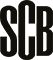Price base amount for 2022

# Price base amount for 2022

Statistical news from Statistics Sweden 2021-07-14 9.30

The price base amount, as defined by the Social Insurance Code (2010:110), has been calculated at SEK 48 300 for 2022, which is SEK 700 higher than in 2021.

Under the current provisions of the Social Insurance Code, calculations of the price base amount are to be based on the base number 36 396, which is the basis for the 1998 price base amount. This base number is to be multiplied annually by a comparative figure that indicates the relationship between the general price level in June of the year preceding the year to which the price base amount relates and the price level in June 1997.

The Government has commissioned Statistics Sweden to calculate the price base amount based on these regulations. The calculations are to be made based on the change in the Consumer Price Index.

## Calculating the Price Base Amount

For June 1997 and June 2021, the Consumer Price Index with two decimals has been calculated at 257.38 and 341.32 respectively (1980=100). Then, the comparative figure has been calculated as 341.32/257.38 = 1.3261. The base number 36 396 that is used as a basis for calculating the price base amount, was multiplied by the comparative figure 1.3261.

Thus, the price base amount for 2022 amounts to SEK 48 300 (36 396 x 1.3261= 48 300), after rounding to the nearest hundred kronor. This is an increase of SEK 700 compared with the price base amount for 2021.

## Increased Price Base Amount

The Government has also commissioned Statistics Sweden to calculate the increased price base amount used in calculations of pensionable income and crediting of pension points. The increased price base amount is to be calculated in the same manner as the regular price base amount, with the only difference that the base number is 37 144. Thus, the increased price base amount for 2022 amounts to SEK 49 300 (37 144 x 1.3261= 49 300) after rounding to the nearest hundred kronor. This is an increase of SEK 700 compared with the increased price base amount for 2021.

The price base amount and the increased price base amount will be determined by the Government.

Feel free to use the facts from this statistical news but remember to state Source: Statistics Sweden.

Statistical agency and producer

Statistics Sweden, Price Statistics

Solna strandväg 86
171 54 Solna
E-mail
priser@scb.se

Enquiries

Beatrice Ljung

Telephone
+46 10 479 40 16
E-mail
beatrice.ljung@scb.se

Sofie Öhman

Telephone
+46 10 479 42 58
E-mail
sofie.ohman@scb.se